cost: \$0

build time: 10 minutes (MVP)

updated: 2021-06-23

Google AppScript is a custom Javascript 'platform' that allows you to write custom scripts for Google Sheets, Docs, Slides, Forms, and Gmail. It is particularly useful for enabling technical functionality like API calls to end users in a UX that is familiar to them.

### #1 - getting started

you can access the script.google.com editor from any sheet. Scripts you save there can be shared across sheets. (You can see in the below image the `~ Tools ~` dropdown menu I've added)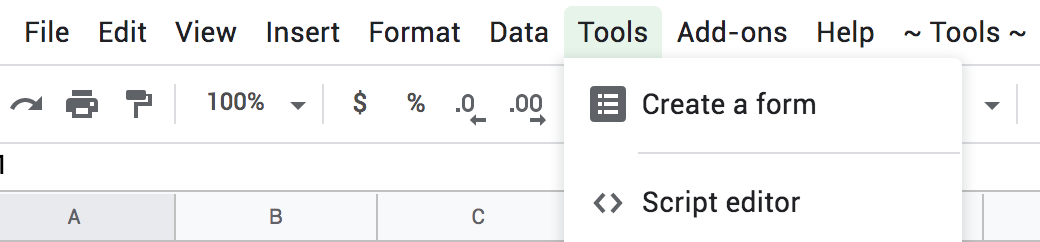you can access the Active Spreadsheet or Sheet (which I will from now on refer to as Tab) at any time.

``````var ss = SpreadsheetApp.getActiveSpreadsheet();

var specificTab = ss.getSheetByName('Results');
``````

### #2 - reading GSheet tabs

#2.1 - get the value of a specific cell

``````var firstValue = tab.getRange("A1").getValue();
``````

#2.2 - get the (rectangular) range where data is present (and their values)

``````var range = sheet.getDataRange();
var values = range.getValues();
``````

#2.3 - get a specific (rectangular) range of cells' values.

``````// the syntax is getSheetValues(start_row, start_col, number_of_rows, number_of_cols).
var range = tab.getSheetValues(1, 3, lastRow, 3);
``````

note: start_row and start_col start at 1, not 0 note #2: number_of_rows/cols is inclusive of the start_row/col (e.g. a number_of_rows=1 will only include the start_row)

#2.4 - get the last row (or column) with values in one or more cells

``````// Remember, this is an index. Add .getValues() to get the values
var lastRow = tab.getLastRow();
var lastCol = tab.getLastColumn();
``````

#2.5 - get every value in a specific column (let's say third from left)

``````var range = tab.getRange(1, 3, lastRow, 1);
var values = range.getValues();
``````

#2.5.1 - great, let's do something with those values. For example, let's log them.

``````for (var i = 0; values.length > i; i++) {
Logger.log(values[i]);
}
``````

#2.6 - get a list of all the Column Headers

``````var columns = tab.getRange(1,1,1, tab.getMaxColumns()).getValues();
``````

#2.7 - .getRange().getValues() produces a list of lists, even for one row. if you want a row as a dict, use this (Gist)

``````function rowToDict(tab, rownumber) {
var columns = tab.getRange(1,1,1, tab.getMaxColumns()).getValues();
var data = tab.getDataRange().getValues()[rownumber-1];
var dict_data = {};
for (var keys in columns) {
var key = columns[keys];
dict_data[key] = data[keys];
}
return dict_data;
}
``````

#2.8 - get a collection of non-adjacent ranges? Docs

``````var rangeList  = sheet.getRangeList(['A1:D4', 'F1:H4']);
listOfRanges = rangeList.getRanges()
``````

### #3 - writing GSheet tabs

#3.1 - overwrite a specific cell

``````SpreadsheetApp.getSheetByName("Data Tab").getRange('F2').setValue('Hello');
``````

#3.2 - append a row at the bottom of the populated rows. (e.g. this will be at the top, if you `.clear()` it first)

``````specificTab.appendRow(["Column A", "Column 2", "Potatoes"]);
``````

#3.2.1 - append a range to the bottom of the populated cells

``````var range_to_write = [[1,"a"],[2,"b"],[3, null]]
tab.getRange(tab.getLastRow()+1, 1, range_to_write.length, range_to_write.length).setValues(range_to_write);
``````

#3.3 - if you truly want to append at the very bottom of a tab, use this instead

``````var row_to_write = [["orange", 3, null]]
tab.getRange(tab.getMaxRows()+1, 1, row_to_write.length, row_to_write.length).setValues(row_to_write);
``````

#3.4 - imagine you want to make an API call for each populated cell in a column, and then write the output to a new tab:

``````for (var i = 1; i < lastRow+1; i++) {
var inputValue = inputTab.getRange(i,1).getValue();
if (inputValue != "" && inputValue !='HEADERVALUE') {
try{
var url = 'https://www.someapithatyouuse.com?q=' + inputValue
var response = UrlFetchApp.fetch(url);
var data = JSON.parse(response.getContentText());
var handled_data = helper_function_you_wrote(data, inputValue);
outputTab.appendRow([
handled_data['Key1'],
handled_data['Key2'],
handled_data['SomeList'].join(", "),
]);
} catch(e){
ss.toast("You have an error with query: " + inputValue + " in row: " + i, "Error", 5)
};
}
}
``````

#3.5 - another pattern is to read from Col A and write to Cols B (C,D,E, etc).

``````// modify the for loop to check that the cell over is empty
if (query != "" && tab.getRange(i+1,2).getValue() == "") {
// API call goes here
tab.getRange(i+1, 2, handled_data.length, handled_data.length).setValues(handled_data);
}
``````

#3.6 - find and replace text in the sheet itself

``````var textFinder = sheet.createTextFinder('input phrase').matchCase(false);
var firstOccurrence = textFinder.findNext();
var numOccurrencesReplaced = firstOccurrence.replaceWith('cat');
``````

#3.7 - sort the resulting tab (in this example, by the first col, descending)

``````tab.sort(1, false);
``````

#3.8 - delete every value on a tab

``````specificTab.clear();
``````

### #4 - API requests

API requests in AppScript are a little weird, as you have to use UrlFetchApp

#4.1 - The base of every call is the same:

``````var response = UrlFetchApp.fetch(url, options);
var status_code = JSON.parse(response.getResponseCode());
var data = JSON.parse(response.getContentText());
``````

#4.2 - GET's

You can convert a dictionary (data_dict)into querystrings easily

``````var querystrings = Object.keys(data_dict).map(function(k) {
return encodeURIComponent(k) + "=" + encodeURIComponent(data_dict[k]);
}).join('&')

url = url + "?" + querystrings
``````

#4.2.1 - want to pass headers (e.g. to add an Auth key)?

``````const API_KEY = PropertiesService.getScriptProperties().getProperty('API_KEY');
var options = {
"method" : "GET",
}
var response = UrlFetchApp.fetch(url, options);
``````

#4.3 - POST's

POSTs are a little more complicated, but operate in a similar manner:

``````var data = {
};
var options = {
"method" : "POST",
"contentType": "multipart/form-data",
"accept": "application/json",
};
var response = UrlFetchApp.fetch(url, options);
``````

if you provide a `payload`, UrlFetchApp will infer the call is a `POST`, even if you specify `GET`

### #5 - end user UX

#5.1 - let's say you want to make a tool available to end users. Just make a menu, with options to invoke specific functions:

``````function onOpen() {
options = [
{name:"Button_1", functionName:"test2"},
];
}
``````

#5.2 - a popup box that the user has to acknowledge?

``````var UI = SpreadsheetApp.getUi();
UI.alert('Here is the value: '+ value);
``````

#5.2 - a popup box disappears after 5 seconds?

``````ss.toast('Body: You have an error with query: ' + query, 'Title: Error', 5)
``````

#5.3 - a popup with an OK and a cancel button, where the user's input drives the script's actions?

``````Browser.msgBox('Check to be sure Column B is empty', Browser.Buttons.OK_CANCEL);
``````

as an example, imagine you wanted to collect a string from the user (e.g. a password). You can do so with this function:

``````function get_data_from_ui_prompt(){
var result = UI.prompt('Put your Secret Key in here', UI.ButtonSet.OK_CANCEL);
var button = result.getSelectedButton();
if (button == UI.Button.OK) {
return result.getResponseText();
// the value is not stored after the session ends
} else if (button == UI.Button.CANCEL) {// User hit "Cancel"
} else if (button == UI.Button.CLOSE) { // User hit the X in the title bar.
}
}
``````

### #6 - gotchas

#6.1 - there are two similar named functions - `SetValue` and `SetValues`.

• Range.setValue() is used for setting the same value in every cell of the range,
• Range.setValues() is used to set an array of values into the corresponding cells in the range.

#6.2 - when you make changes to the to the SS Dropdown menu, you have to reload sheet (and exit code editor) to see them take effect.

#6.3 - `Ljava.lang.Object` is the AppScript equivalent of `[object Object]`

#6.4 - there are a few different Log interfaces. Google doesn't explain them well. Docs are here, but suffice to say you should just use

``````Logger.log("thing you want written");
// and then check logs with CMD+Enter in the script.google.com editor
``````

#6.5 - make sure you're encoding your URL components correctly:

``````function encode_url_component(query) {
query = query.replace(/^\s+|\s+\$/g, '') // Trim left and right whitespace
query = query.replace("%", "%25").replace("#", "%23").replace("|", "%7C").replace('"', "").replace('"', "").replace("'", "").replace("'", "").replace("&", "%26");
return query
}
``````

#6.6 - you can chose to mute HTTP exceptions, so the user doesn’t see / the script isn’t interrupted by 404s (SO)

``````var response = UrlFetchApp.fetch(url,{muteHttpExceptions: true});
``````

### #7 - snippets

#7.1 - set Environment Variable (you have to run SetKeys once)

``````function SetKeys(){
PropertiesService.getScriptProperties().setProperty('SOME_KEY', 'o1QVl6EG7FxprvBxbqvm');
}
``````

fetch that key later

``````SOME_KEY = PropertiesService.getScriptProperties().getProperty('SOME_KEY');
``````

#7.2 - get the current datetime as a string

``````var timestamp = new Date().toLocaleString();
``````

#7.3 - get the color of a range of cells:

``````var range = SpreadsheetApp.getActiveRange();
var color = range.getBackgroundColor();
``````

#7.4 - copy a tab (can be to same GSheet or to another one)

``````var destination = SpreadsheetApp.openById('ID_GOES HERE');
tab.copyTo(destination);
``````

#7.5 - resize the first n (e.g. 15) columns to fit the text in the cells of that column

``````tab.autoResizeColumns(1, 15);
``````

#7.6 - hide and unhide tabs

``````tab.hideSheet();
tab.showSheet();
``````

#7.7 - freeze and unfreeze rows and columns (in this case, the first row/col)

``````sheet.setFrozenRows(1);
sheet.setFrozenColumns(1);
``````

### #8 - auth for sharing AppScript

as of 2021, you must jump through some hoops to have other people use AppScript code that you wrote.

in order to allow access to other users, you must create a GCP Project and associate it with the AppScript file:

#8.1. get the necessary AppScript OAuth Scopes from the AppScript file: File -> Project properties -> Scopes

#8.2. go to the Google Cloud Platform console and create a new Project - https://console.cloud.google.com/projectcreate

#8.3. ’switch’ to the newly created Project#8.4. create a OAuth ClientID and Consent Screen: Credentials -> Create Credentials -> OAuth ClientID -> Configure Consent Screen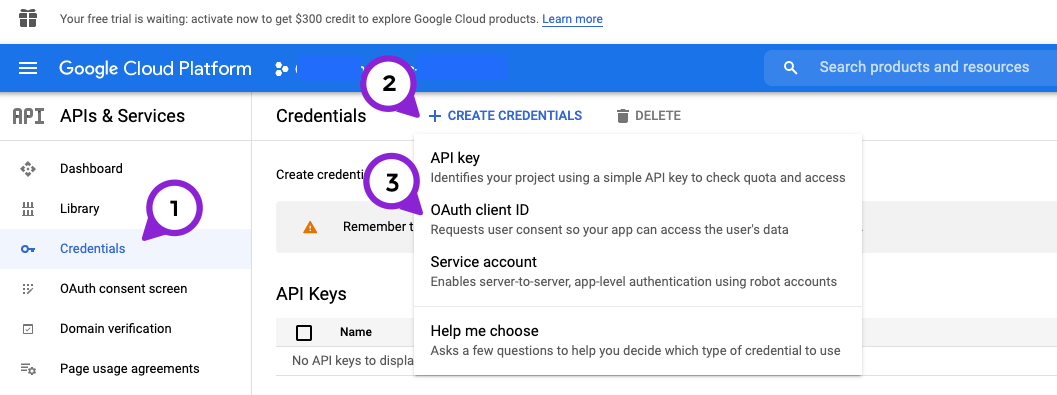pick User Type `External` if users whose emails are not on your domain will use it.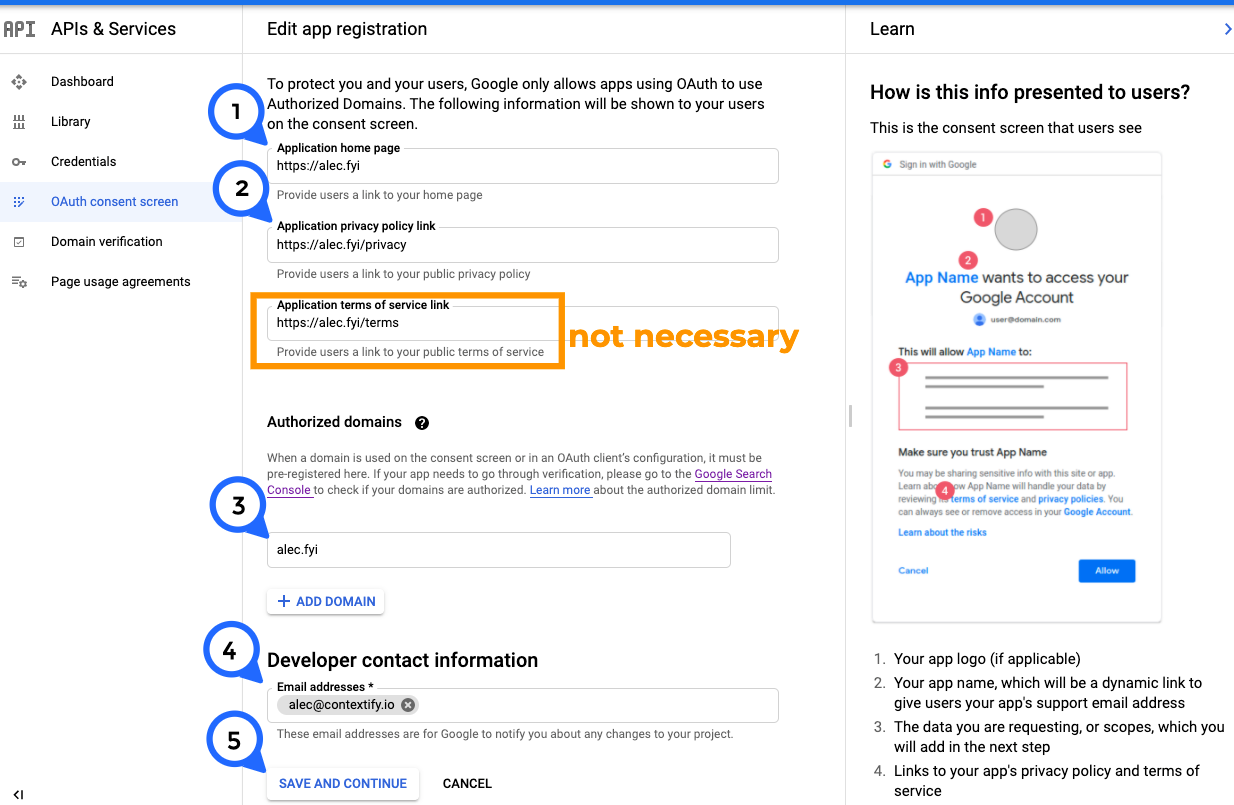#8.5. take the `Scopes` you got from 8.1 and add them, separated by commas: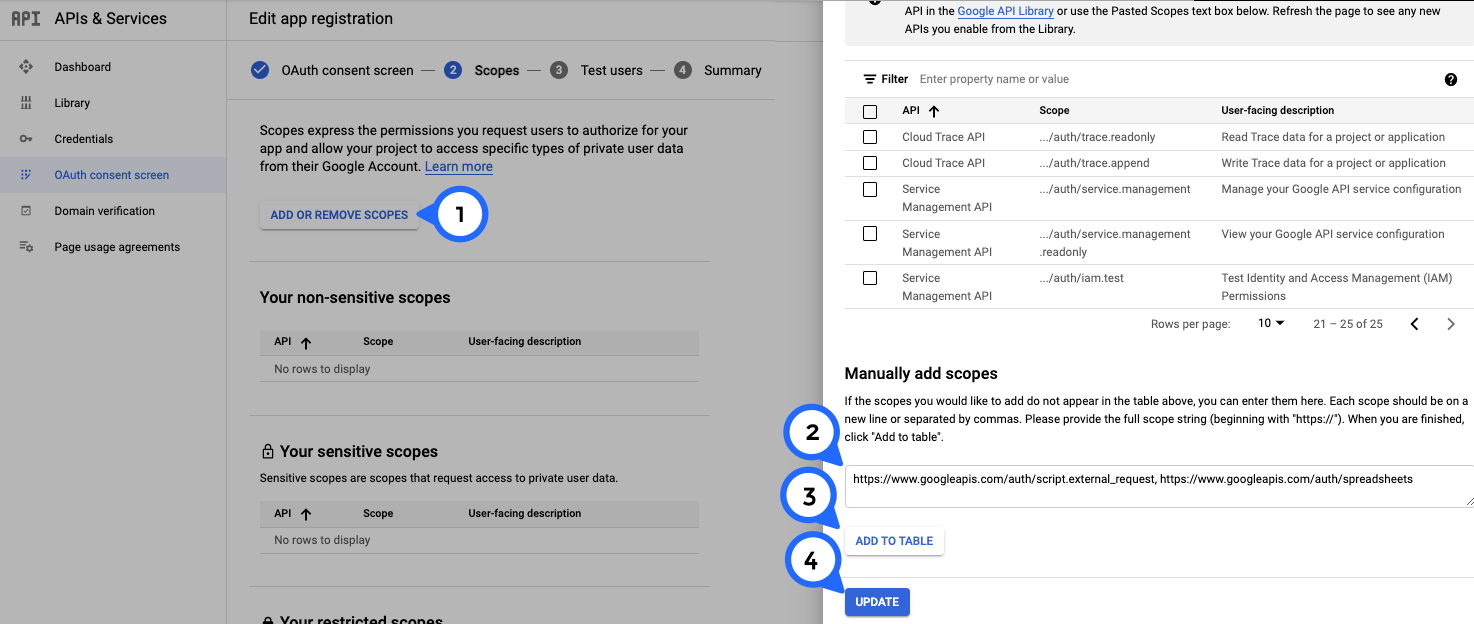#8.6.1 Authorized domains are authorized through Google Search Console for some reason. You'll have to add a TXT record to the DNS settings of your domain

#8.7. add the users as test users (up to 100)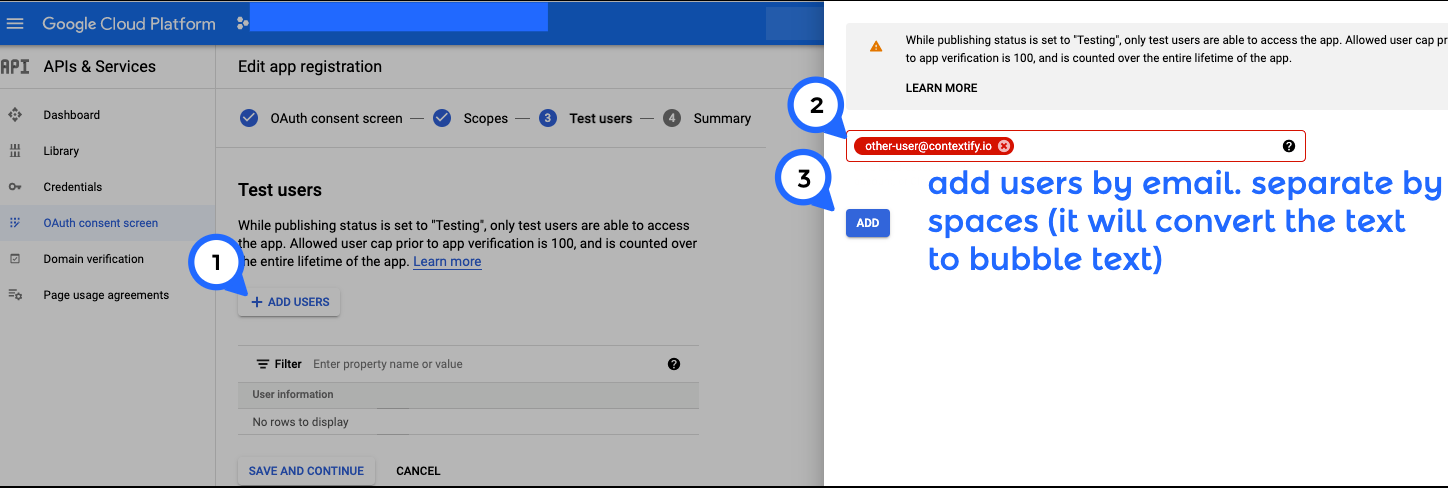#8.8. get your GCP Project ID from the GCP console: ⋮ > Project settings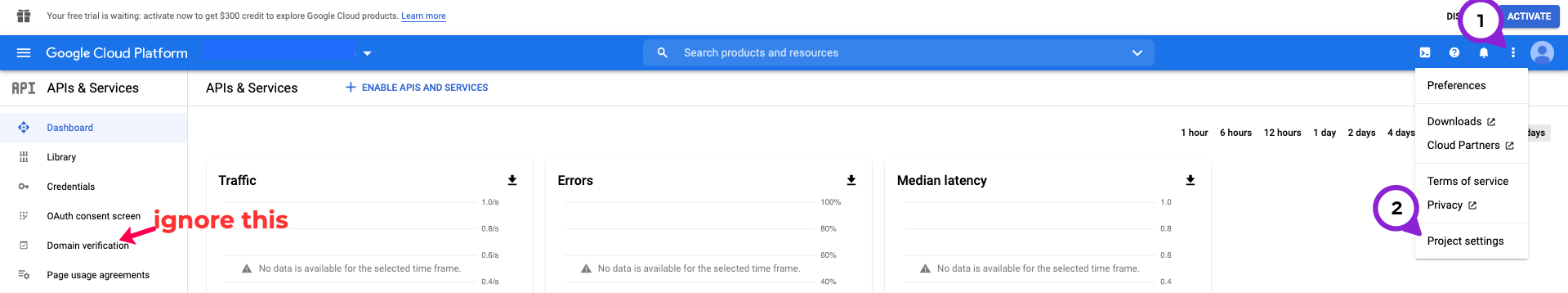keep in mind there is a `Domain verification` tab on the left sidebar. It will inaccurately show no domains verified, even after you verify your domain through its provided modal. You can safely ignore it.

#8.9. associate the GCP Project ID with the AppScript file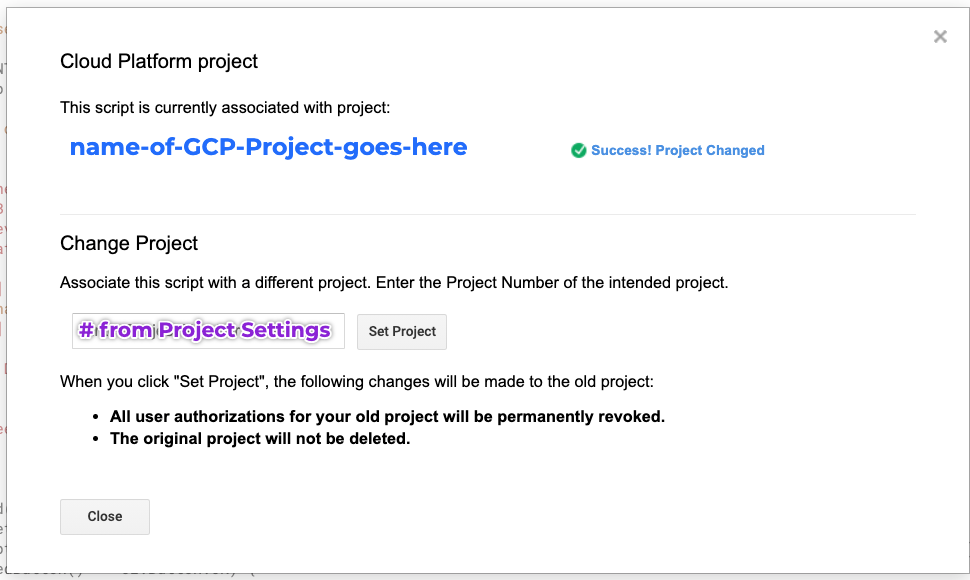#8.10. at some point you should verify the app with Google, but candidly, I could not figure out how after a couple hours of trying